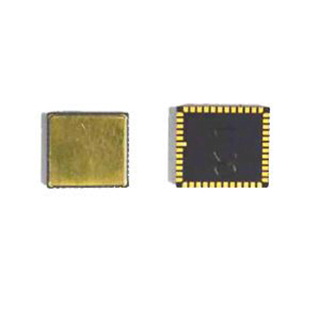Application

# Analysis of the main performance parameters of MEMS gyroscopeRange (DYNANMIC RANGE)

Sensitivity

Zero angular velocity output

INTER AXIS sensitivity

scale factor

Nonlinearity

Linear acceleration sensitivity

Vibration Sensitivity

Zero bias stability

noise density

Angle free walk system

bandwidth

Bias Voltage Sensitivity

Self-check function

Power consumption

shock survivability

range of working temperature

Packaging error

The range of the gyroscope is usually expressed by the maximum value of the input angular rate in the forward and reverse directions, for example: +/-300 degree/sec. The larger the value, the stronger the ability of the gyroscope to be sensitive to the angular rate. Within this input angular rate range, the nonlinearity of the gyroscope scale factor can meet the specified requirements, and the range of the gyroscope can usually be configured. ER-MG2-300/400 can up to ±400°/s measurement range.

Sensitivity (resolution) represents the minimum perceptible input angular rate increment at a specified input angular rate, eg 0.05 degree/sec/LSB. Generally speaking, the larger the measurement range of a MEMS gyroscope, the corresponding reduction in sensitivity.

The scale factor (scale factor) is the ratio of the output of the gyroscope to the angular rate of the input. This ratio is represented by the slope of a specific straight line, which is obtained by least squares fitting based on the input and output data measured over the entire input angular rate range.

The nonlinearity is the ratio of the maximum deviation of the gyroscope output relative to the least squares fitting straight line to the maximum output within the range of the input angular rate, which characterizes the deviation of the actual input and output data of the gyroscope and determines the fitting reliability of the data.

Linear acceleration sensitivity reflects the sensitivity of the gyroscope to acceleration, and the unit is degree/sec/g.

Vibration sensitivity refers to the sensitivity of the gyroscope to vibration, and the unit is degree/sec/g2. The less sensitive the gyroscope is to linear accelerations and vibrations, the better the performance of the gyroscope and the more efficient the algorithm that can be built.

Zero bias, also known as the accuracy of the gyroscope, refers to the output of the gyroscope in the zero input state. It is represented by the equivalent conversion of the mean value of the output over a longer period of time to the input angular rate, that is, the degree of dispersion of the observed value around the zero deviation. For example, 0.005 degree/sec means that the deviation will be 0.005 degree per second. The long-term steady-state output in the zero-input state is a stationary random process, that is, the steady-state output will fluctuate and fluctuate around the mean (zero-bias), which is customarily represented by the mean square error, which is defined as the zero-bias stability. The initial zero bias error can be understood as a static error, which will not fluctuate with time and can be calibrated by software. Ericco’s ER-MG2-100 and ER-MG2-300/400 are currently ERICCO’s highest precision MEMS gyroscopes, and their bias stability (Allan variance) is only 0.02°/h and 0.03°/h.

When the gyroscope is in the zero input state, the shedding output signal is the superposition of white noise and slowly varying random functions. Diffuse random functions can be used to determine bias and bias stability metrics. White noise is defined as the standard deviation of the equivalent rotational angular velocity at the square root of the unit detection bandwidth, in units (degree/sec/√Hz or degree/hr/√Hz). This white noise can also be represented by an angle random walk coefficient in degrees/√Hz. The random walk coefficient refers to the gyroscope output error coefficient accumulated over time generated by the white noise. When the external conditions are basically unchanged, it can be considered that the main statistical characteristics of the various noises analyzed above do not change with the passage of time.

The bias voltage sensitivity refers to the sensitivity of the output of the gyroscope to the change of the power supply, for example: 0.03degree/sec/V, that is, how much the output angular rate changes for every 1V change of the power supply.

Bandwidth refers to the frequency range over which the gyroscope can accurately measure the input angular rate. The larger the range, the stronger the dynamic response capability of the gyroscope.

The self-test function automatically tests the mechanical and CMOS circuit parts of the device before use to provide system robustness.

The power consumption includes the power consumption when the gyroscope is running at different resolutions or different data output rates, and the sleep power consumption. This indicator is especially important in low-power applications such as wearables and IoT applications.

Shock survivability refers to the ability of the gyroscope to withstand acceleration shocks of different degrees. For example, after a 2000g acceleration shock, the gyroscope ensures the normal operation of the system. Considering that the application environment of the gyroscope may receive a large impact, this indicator is particularly important. Generally, the gyroscope will hang up when the acceleration exceeds its tolerance range, and it must be restarted and powered on before it can work normally.

Range of working temperature—The mechanical structure of the MEMS gyroscope determines that the temperature will affect the data output, and exceeding the operating temperature range may cause a large deviation in the data output.

Package error is the angle between the die diagonal and the package diagonal.